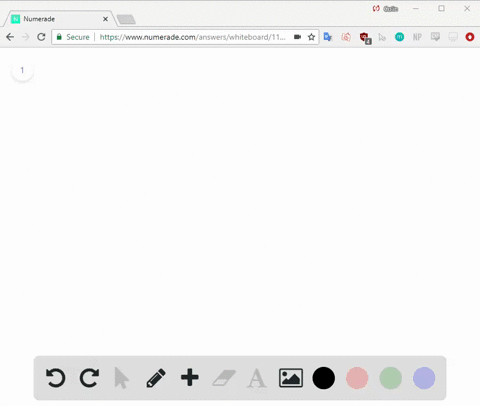### $$y=x^{2}-6 x+8$$ The equation above represents …

01:00Johns Hopkins University
Problem 6

Where Do People Get Most of
Their Medical Information?
$$\begin{array}{|c|c|}\hline {\text { Source }} & {\text { Percent of }} \\ & {\text { those surveyed }} \\ \hline \text { Doctor } & {63 \%} \\ \hline \text { Internet } & {13 \%} \\ \hline \text { Magazines/brochures } & {9 \%} \\ \hline \text { Pharmacy } & {6 \%} \\ \hline \text { Television } & {2 \%} \\ \hline \text { Other/none of the above } & {7 \%} \\ \hline\end{array}$$
The table above shows a summary of $1,200$ responses
to a survey question. Based on the table, how many
of those surveyed get most of their medical
information from either a doctor or the Internet?
$$\begin{array}{l}{\text { A) } 865} \\ {\text { B) } 887} \\ {\text { C) } 912} \\ {\text { D) } 926}\end{array}$$

Check back soon!
SAT
SAT Practice Test # 6

## Discussion

You must be signed in to discuss.

## Video Transcript

So it says this is a summary of twelve hundred responses, and so we want to see based on the table how many of those surveyed got most their medical information from either a doctor or the Internet. So here's the doctor responses in sixty three person Internet is thirteen percent. So if what if we saw his doctor or Internet, we know or means to add it. So we're going to add the percentages for doctor and Internet pretty sixty three percent plus thirteen percent. We're going to get seventy six percent. So then we're going to take that seventy six percent and find what, seventy six percent of twelve hundred? It's twelve hundred times zero point seven six because their point seven six is the same thing at seventy six express a decimal. Then we're going to do that to twelve hundred times. Point seven six was going to be nine hundred and twelve. Some see is our answer